# 数据挖掘05-偏相关分析【原理、案例、python实现】

## 一、需求场景

[En]

As we all know, there are many factors that affect the mileage, including the remaining charge of the battery, battery performance (total discharge voltage of the battery pack, inconsistency of the battery pack, single voltage, internal resistance of the battery pack), vehicle parameters, vehicle load, vehicle driving characteristics and temperature.

## 二、偏相关分析简介

### 2.2 什么是偏相关分析

[En]

The partial correlation coefficient is different from the simple correlation coefficient, so it is necessary to master the data of multiple variables when calculating the partial correlation coefficient.

[En]

On the one hand, we consider the possible effects of multiple variables, on the other hand, we use certain methods to control other variables, specifically to investigate the net correlation between two specific variables.

[En]

In the case of multivariable correlation, there is a complex relationship between variables, so there may be a great difference between the partial phase correlation number and the simple correlation coefficient, and sometimes even the symbol may be opposite. The range of partial correlation coefficient is the same as that of simple correlation coefficient, which is from * -1 to 1cm . The greater the absolute value of partial correlation coefficient * (closer to 1) * , the higher the degree of linear correlation between variables * ; the smaller the absolute value of partial correlation coefficient * (the closer to 0) * , the lower the degree of linear correlation between variables * .

### 2.3 偏相关分析的步骤

[En]

The use of partial phase correlation number to analyze the net relationship between variables usually needs to complete the following two steps:

#### （1）计算样本的偏相关系数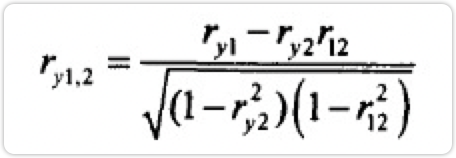ry1、ry2、r12分别表示y和x1的相关系数、y和x2的相关系数、x1和x2的简单相关系数

#### ; （2）对上一步求得的偏相关系数进行检验

1. 提出假设，假设偏相关系数与零无显著性差异，即两个变量之间不线性相关。
2. 选择检验统计量。偏相关分析的检验统计量为t统计量，它的数学定义为：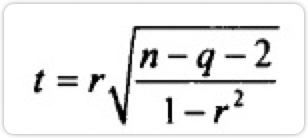r为偏相关系数，n为样本数，q为阶数。t统计量服从n-q-2个自由度的t分布。
3. 计算检验统计量的观测值和对应的概率p值
4. 决策。如果检验统计量的概率p值小于给定的显著性水平α（一般为0.05），应拒绝零假设，认为两总体的偏相关系数与零有显著性差异，即两变量之间有显著的线性相关关系；反之，如果检验统计量的概率p值大于给定的显著性水平α，则说明两变量之间没有显著线性相关关系。

## 三、【案例分析】行驶里程与影响因素的偏相关分析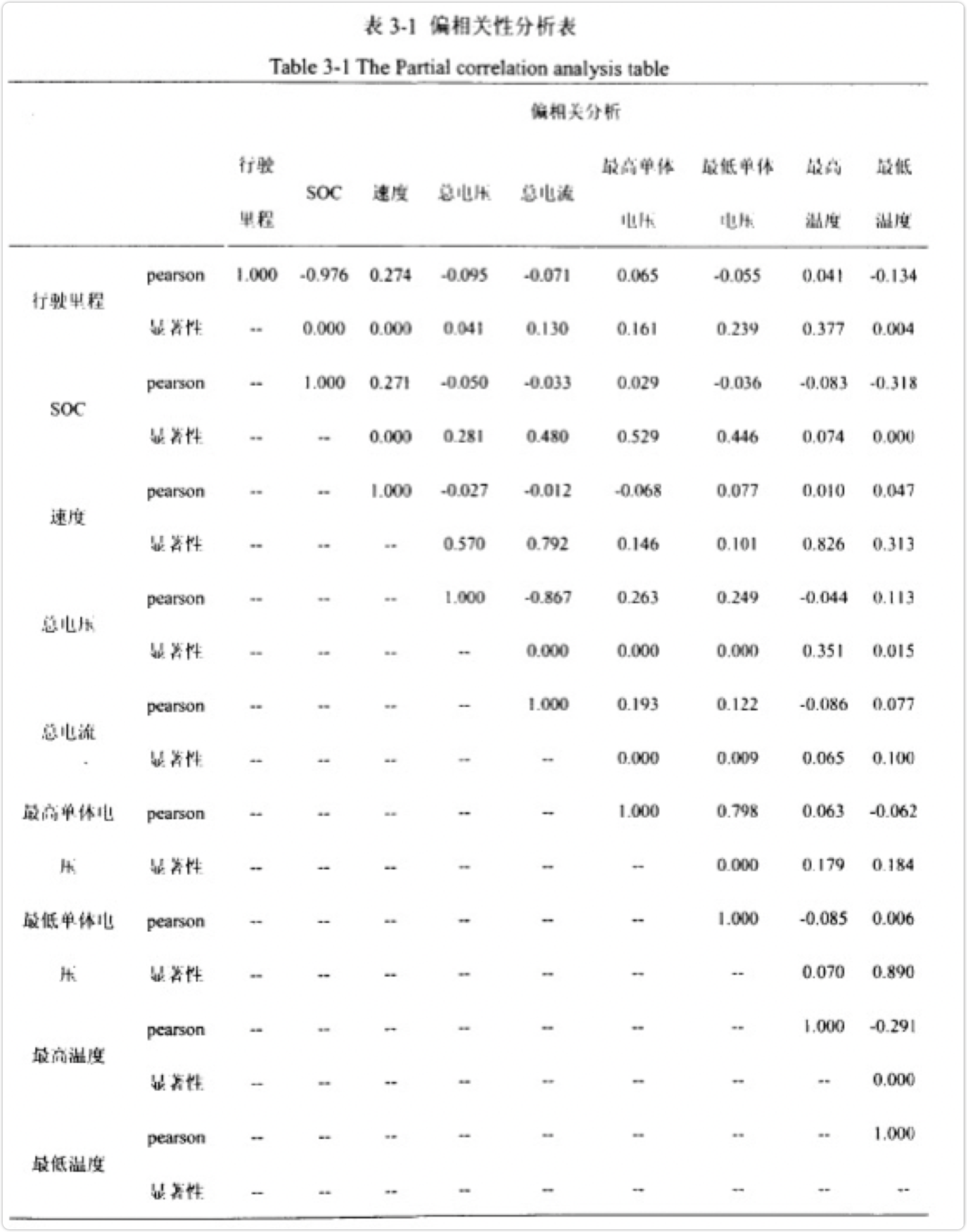### ; 3.1 速度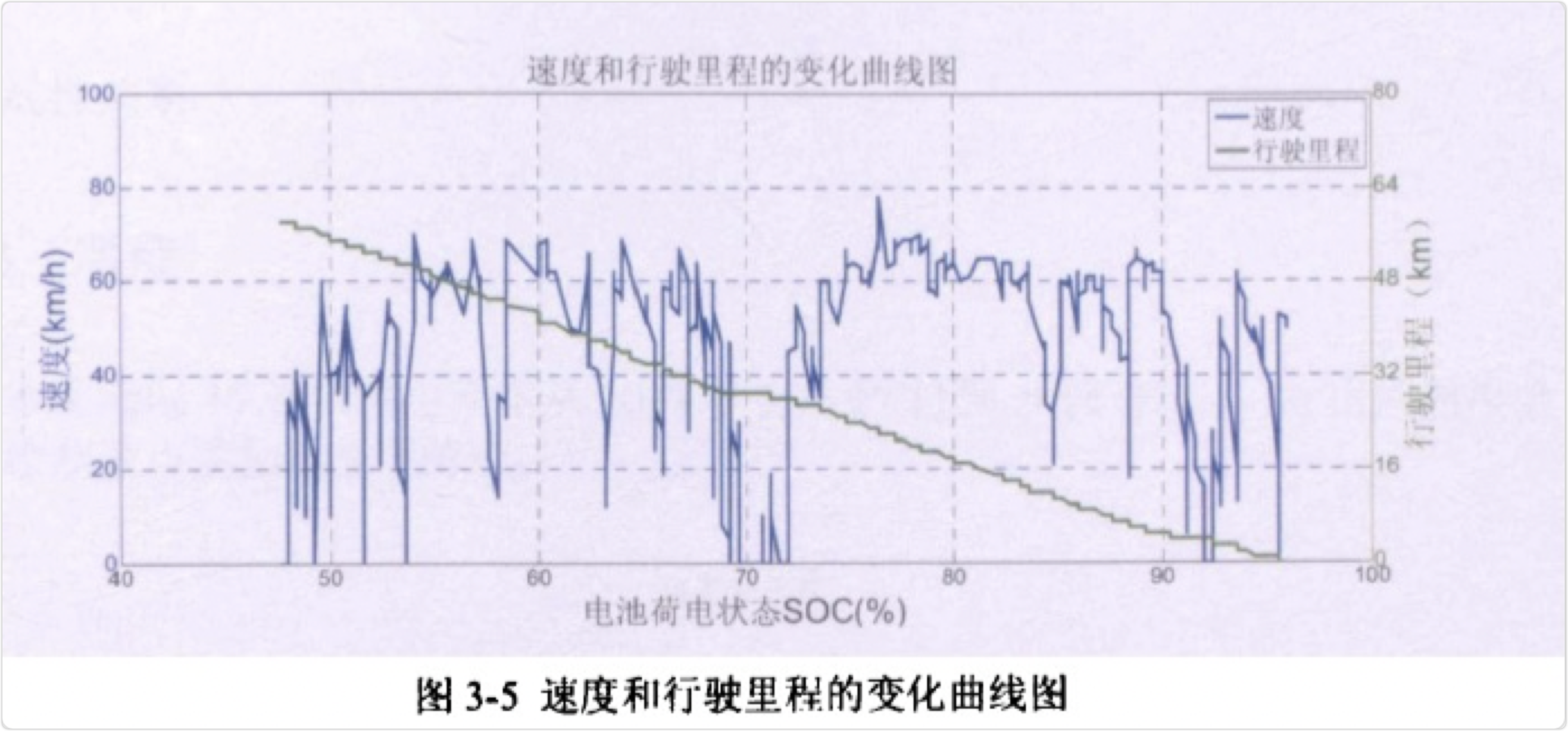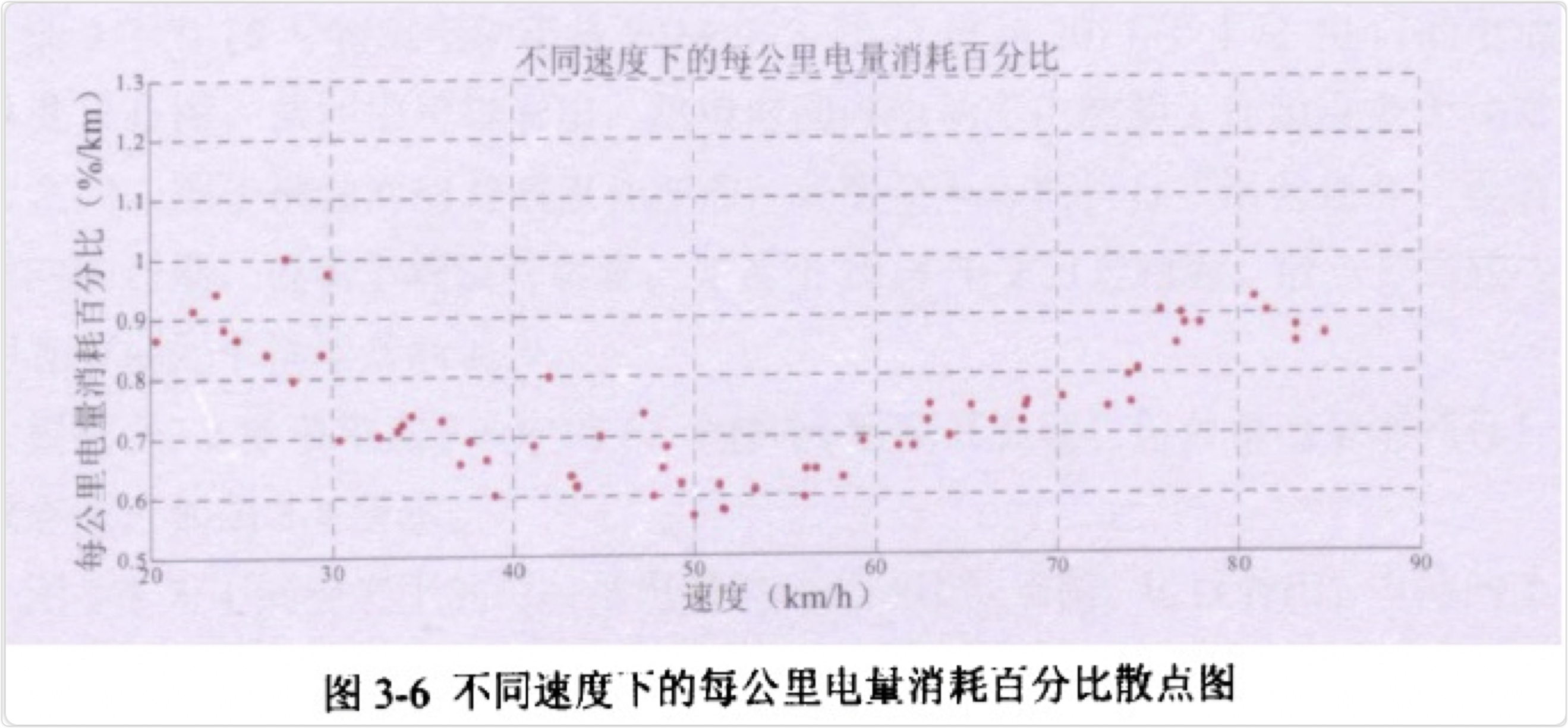### 3.2 温度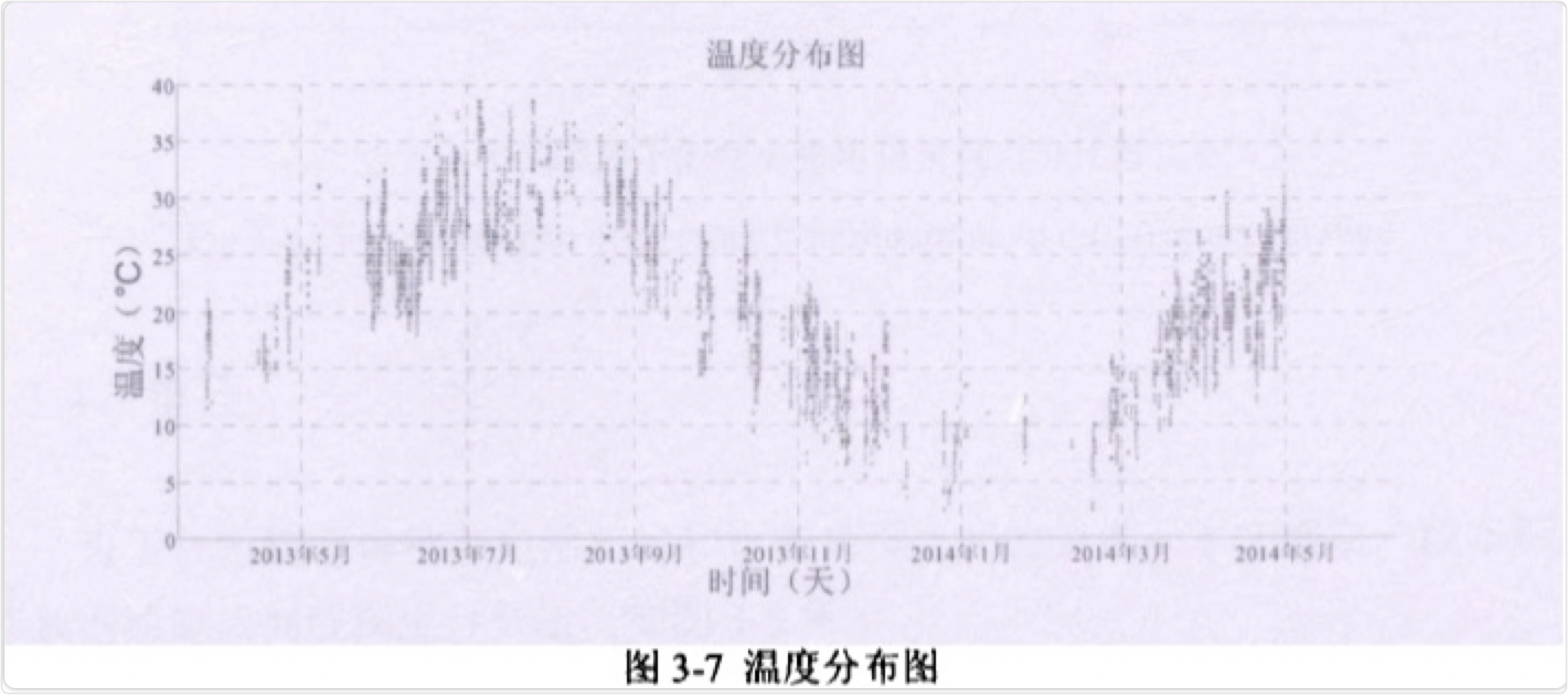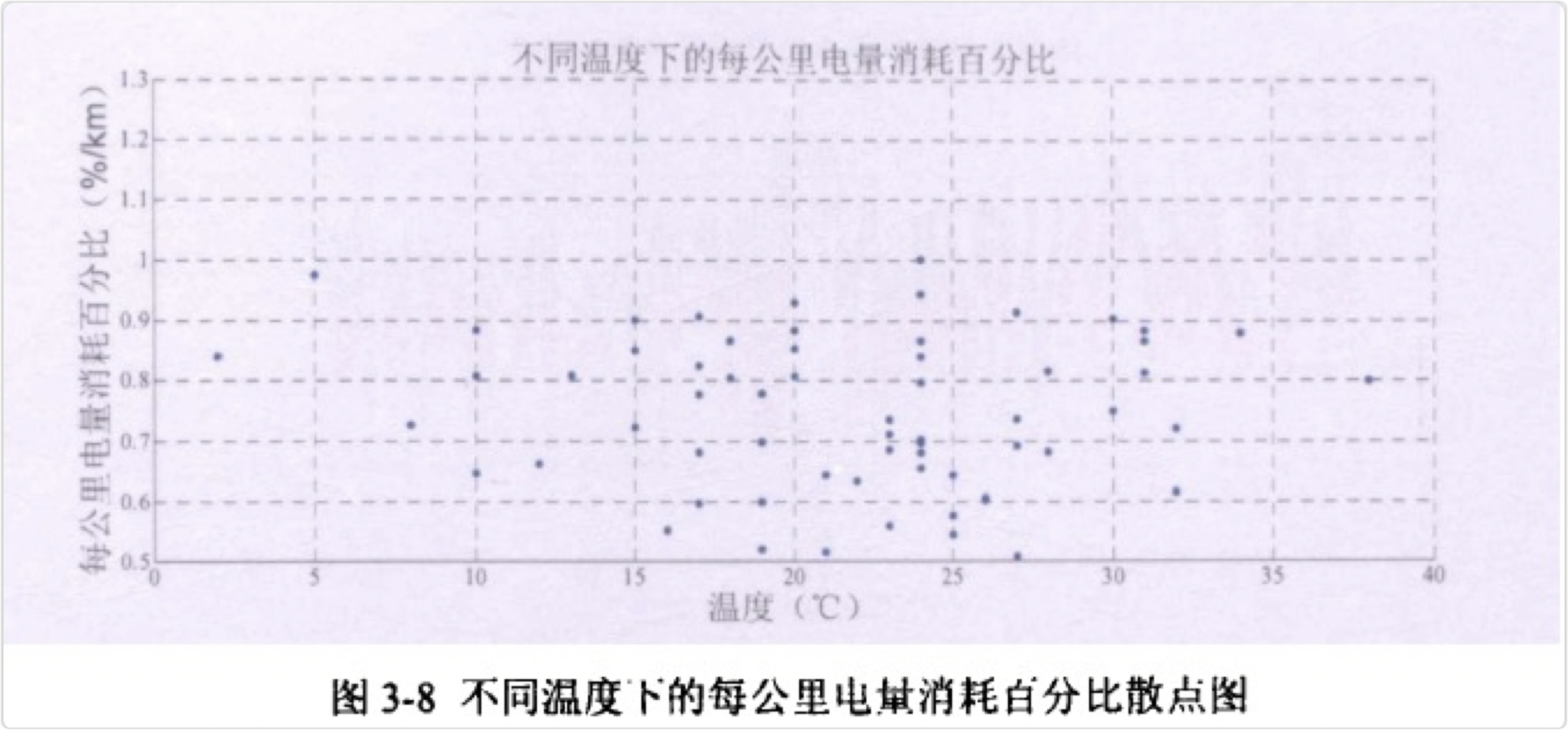### ; 3.3 电压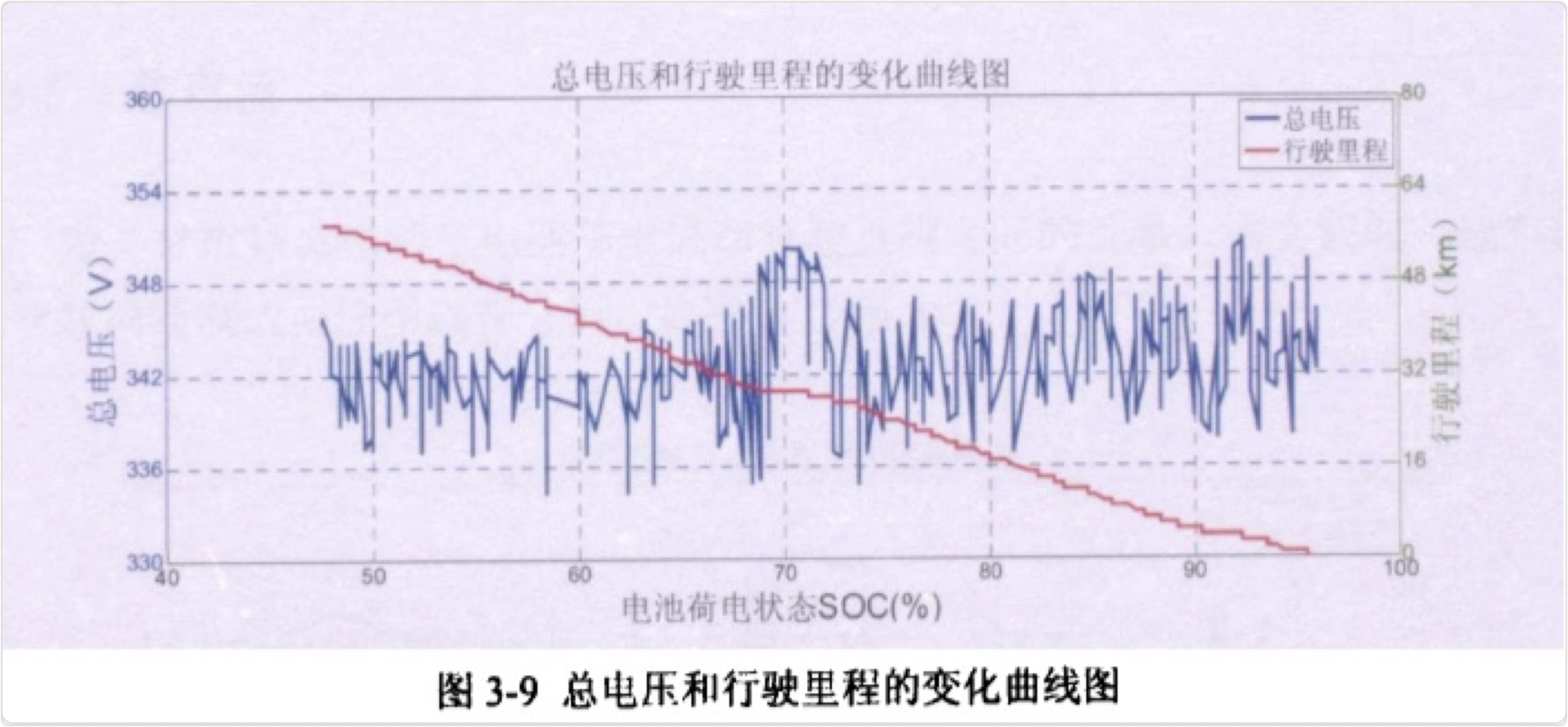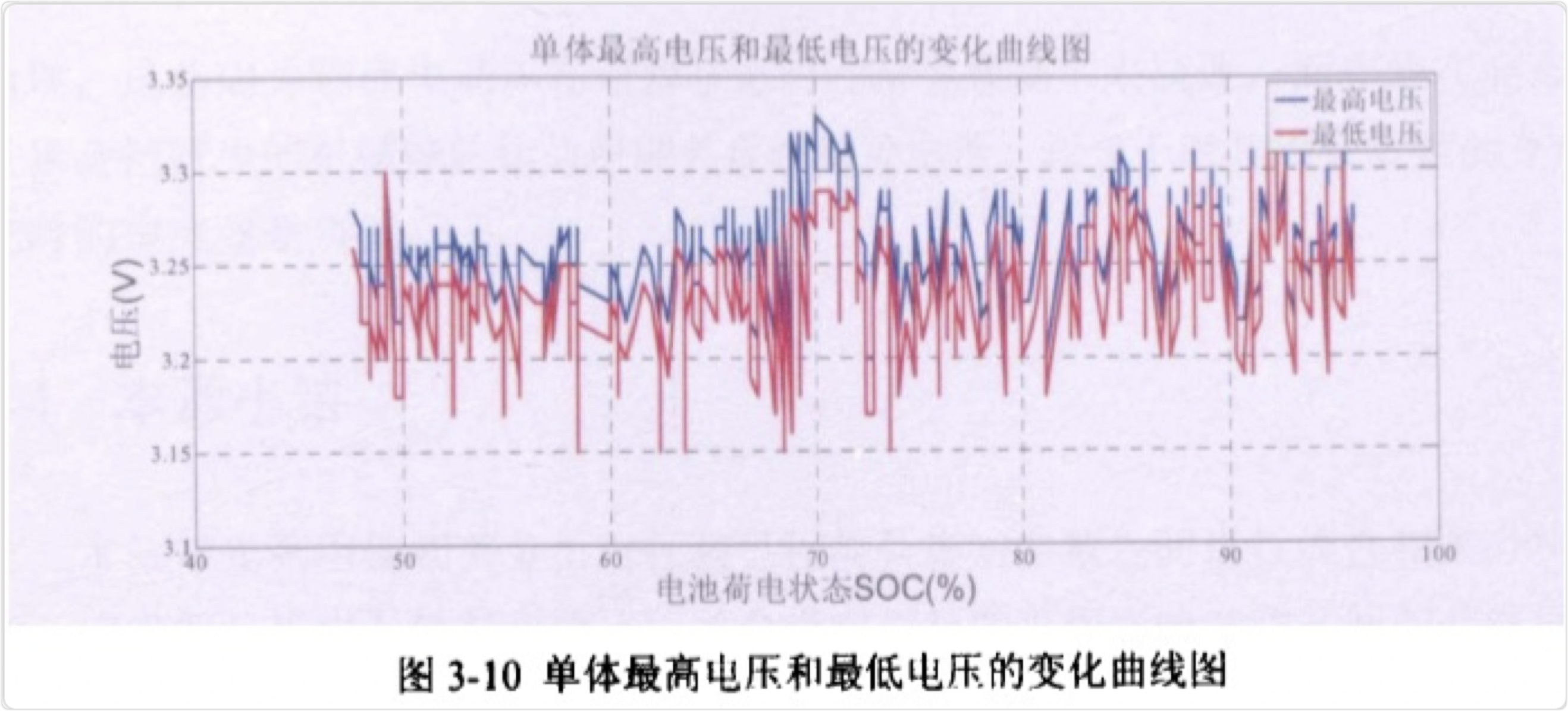### 3.4 总电流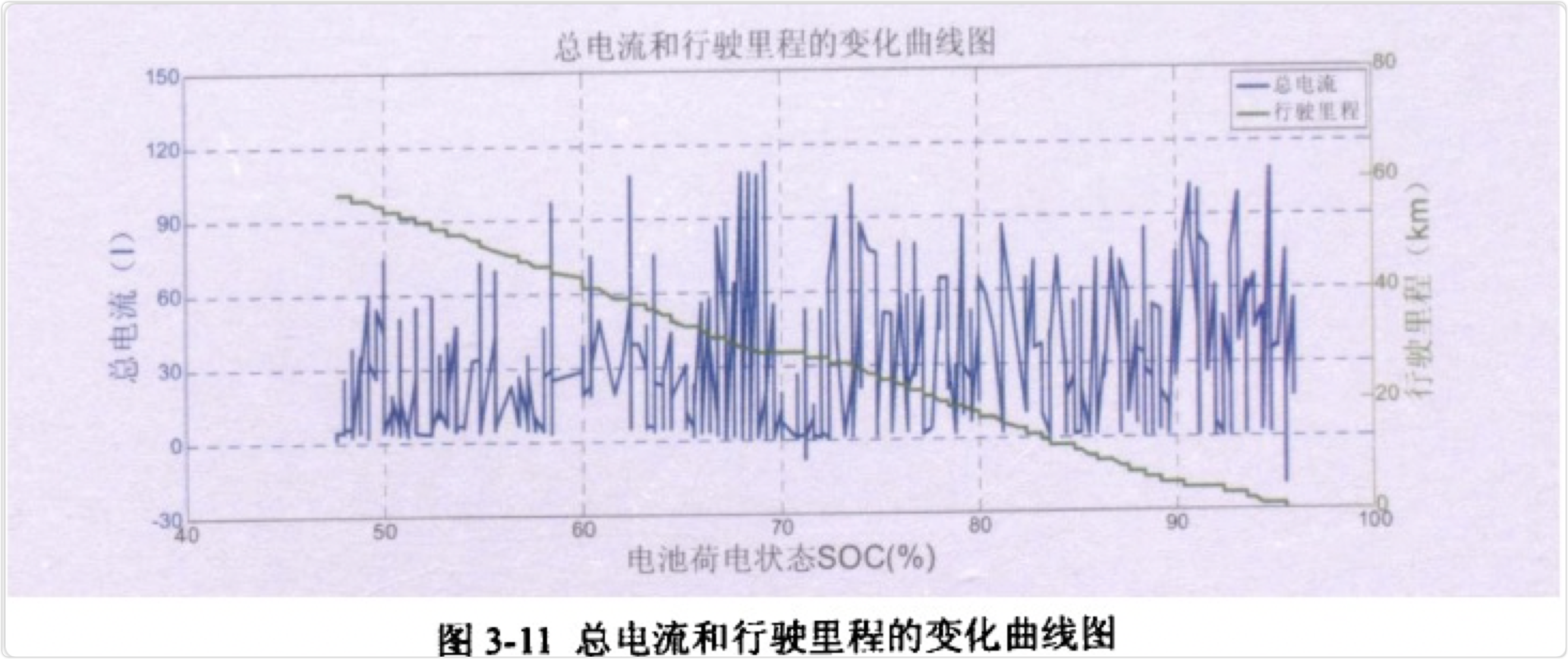## 四、python偏相关分析

### 4.1 数据源

x1=np.random.rand(10)
x2=np.random.rand(10)
x3=np.random.rand(10)
print(x1)
print(x2)
print(x3)


### 4.2 pandas

#### 4.2.1 一阶相关系数


df=pd.DataFrame([x1,x2,x3],index=['a','b','c']).T
print('数据:\n',df)
print('相关系数矩阵为：\n',df.corr())
r_ab=df.a.corr(df.b)
r_ac=df.a.corr(df.c)
r_bc=df.b.corr(df.c)
r_ab_c=(r_ab-r_ac*r_bc)/(((1-r_ac**2)**0.5)*((1-r_bc**2)**0.5))
print('ab_c的一阶偏相关系数为：',r_ab_c)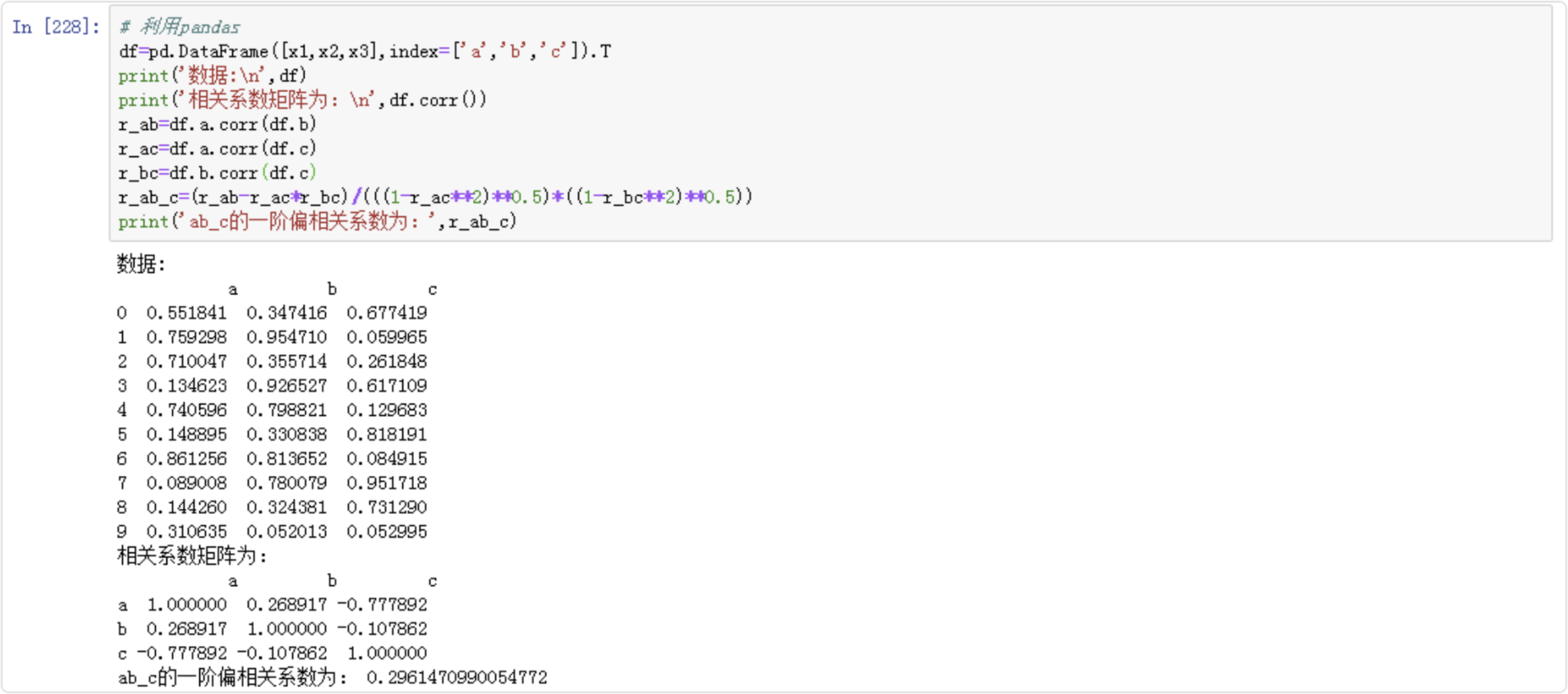#### 4.2.2 pcorr()


df.pcorr().round(3)

hours              -0.311   1.000       0.191
examScore       0.736   0.191       1.000


### 4.3 numpy


lst=[x1,x2,x3]
res=np.corrcoef(lst)
print('相关系数矩阵为：\n',res)
label=['a','b','c']
corr=dict()
for row in range(res.shape):
for col in range(res.shape):
corr['r_{}{}'.format(label[row],label[col])]=res[row][col]
r_ab=corr['r_ab']
r_ac=corr['r_ac']
r_bc=corr['r_bc']
r_ab_c=(r_ab-r_ac*r_bc)/(((1-r_ac**2)**0.5)*((1-r_bc**2)**0.5))
print('ab_c的一阶偏相关系数为：',r_ab_c)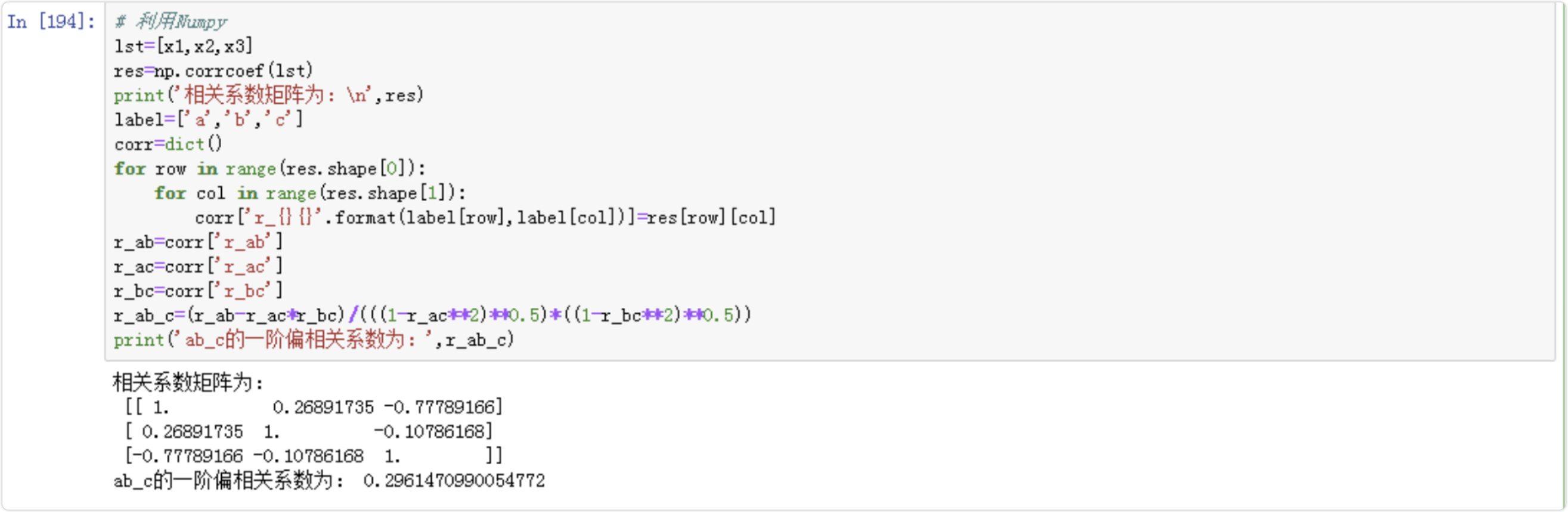### 4.4 sicpy


from scipy import stats
r_ab=stats.pearsonr(x1,x2)
r_ac=stats.pearsonr(x1,x3)
r_bc=stats.pearsonr(x2,x3)
r_ab_c=(r_ab-r_ac*r_bc)/(((1-r_ac**2)**0.5)*((1-r_bc**2)**0.5))
print('ab_c的一阶偏相关系数为：',r_ab_c)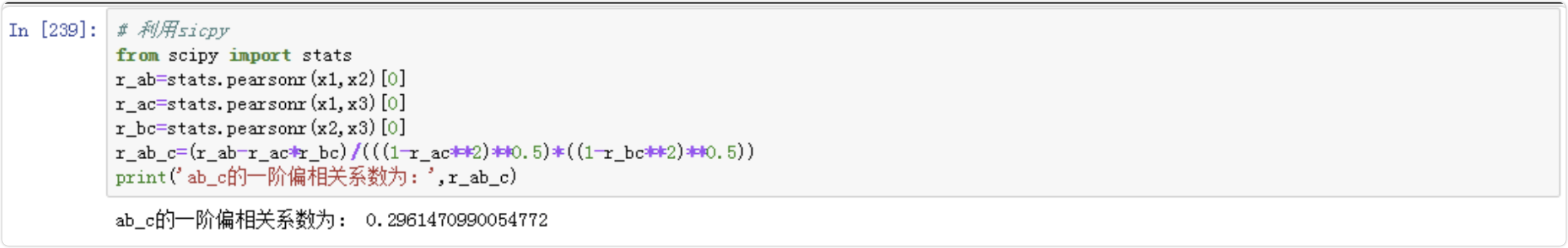### 4.5 自己写公式


import math
def calc_corr(a,b):
E_a = np.mean(a)
E_b = np.mean(b)
E_ab=np.mean(list(map(lambda x:x*x,zip(a,b))))

cov_ab = E_ab - E_a * E_b

def square(lst):
res=list(map(lambda x:x**2,lst))
return res

D_a=np.mean(square(a))-E_a**2
D_b=np.mean(square(b))-E_b**2

σ_a=np.sqrt(D_a)
σ_b=np.sqrt(D_b)

corr_factor = cov_ab / (σ_a*σ_b)
return corr_factor

r_ab=calc_corr(x1,x2)
r_ac=calc_corr(x1,x3)
r_bc=calc_corr(x2,x3)
r_ab_c=(r_ab-r_ac*r_bc)/(((1-r_ac**2)**0.5)*((1-r_bc**2)**0.5))
print('ab_c的一阶偏相关系数为：',r_ab_c)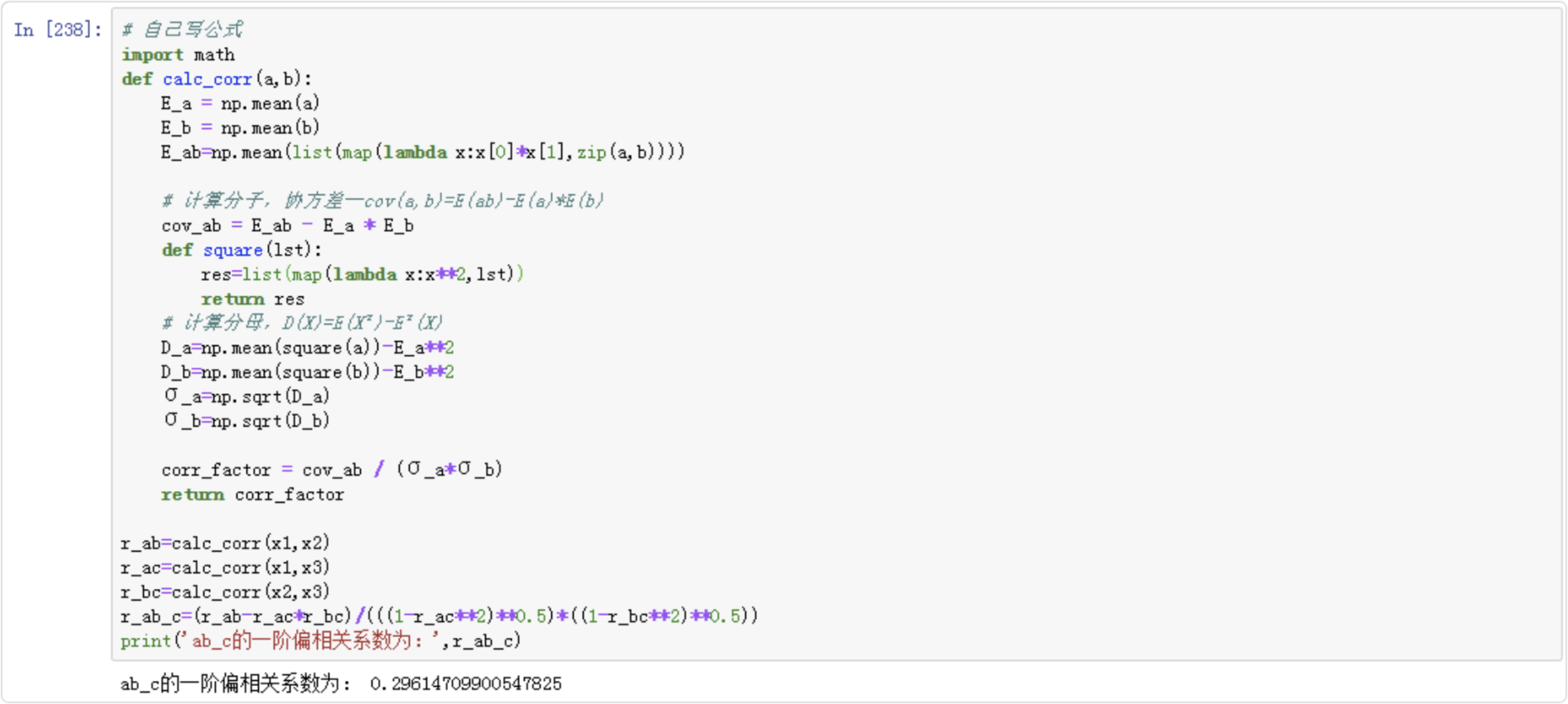Original: https://blog.csdn.net/SeafyLiang/article/details/121667457
Author: SeafyLiang
Title: 数据挖掘05-偏相关分析【原理、案例、python实现】

## 自注意力机制(Self-attention)

Transformer 的处理方式有所不同。它会一次读取所有单词，并权衡每个单词的重要性。因此，更多的注意力集中在更重要的单词上，因此也称为注意力。

### 计算机视觉中的自注意力

1. 值 (value) 表示输入特征。我们不希望自注意力模块查看每个像素，因为这在计算上过于昂贵且不必要。相反，我们对输入激活的局部区域更感兴趣。因此，值在激活图尺寸（例如，它可以被下采样以具有较小的高度和宽度）和通道的数目方面都减小了来自输入特征的维数。 对于卷积层激活，通过使用1x1卷积来减少通道数，并通过最大池化或平均池化来减小空间大小。
2. 键和查询 (Keys and queries) 用于计算自注意图中特征的重要性。为了计算位置x x x 处的输出特征，我们在位置x x x 处进行查询，并将其与所有位置处的键进行比较。
为了进一步说明这一点，假设我们有一幅肖像，当网络处理该肖像的一只眼睛时，它首先进行具有语义意义的查询，并使用该肖像的其他区域中的关键字来检查它。如果其他区域中的一个关键是眼睛，那么网络知道它找到了另一只眼睛，这是网络应该关注的区域，以便网络可以进一步处理它。

[En]

To further illustrate this point, suppose we have a portrait that, when the network processes one eye of the portrait, it first makes a query that has the semantic meaning of "eye" and checks it using keys in other areas of the portrait. If one of the keys in other areas is the eye, then the network knows that it has found the other eye, which is the area that the network should pay attention to so that the network can process it further.

更一般的，使用数学方程表达：对于特征0 0 0，我们计算向量q 0 × k 0 , q 0 × k 1 , q 0 × k 2 , q 0 × k N − 1 q_0×k_0, q_0×k_1, q_0×k_2, q_0×k_{N-1}q 0 ​×k 0 ​,q 0 ​×k 1 ​,q 0 ​×k 2 ​,q 0 ​×k N −1 ​。然后使用s o f t m a x softmax s o f t m a x 将向量归一化，因此它们的总和为 \$1.0，这就是是我们要求的注意力得分。将注意力得分用作权重以执行值的逐元素乘法，以获取注意力输出。## ; Tensorflow实现自注意力模块

class SelfAttention(Layer):
def __init__(self):
super(SelfAttention, self).__init__()

def build(self, input_shape):
n,h,w,c = input_shape
self.n_feats = h * w
self.conv_theta = Conv2D(c//8, 1, padding='same', kernel_constraint=SpectralNorm(), name='Conv_Theta')
self.conv_phi = Conv2D(c//8, 1, padding='same', kernel_constraint=SpectralNorm(), name='Conv_Phi')
self.conv_g = Conv2D(c//8, 1, padding='same', kernel_constraint=SpectralNorm(), name='Conv_g')
self.conv_attn_g = Conv2D(c//8, 1, padding='same', kernel_constraint=SpectralNorm(), name='Conv_AttnG')
self.sigma = self.add_weight(shape=, initializer='zeros', trainable=True, name='sigma')


1. 内部的激活可以减小尺寸，以使计算运行更快。
2. 在每个卷积层之后，激活由形状 (H, W, C) 被重塑为形状为 (H*W, C) 的二维矩阵。然后，我们可以在矩阵上使用矩阵乘法。

    def call(self, x):
n, h, w, c = x.shape
theta = self.conv_theta(x)
theta = tf.reshape(theta, (-1, self.n_feats, theta.shape[-1]))
phi = self.conv_phi(x)
phi = tf.nn.max_pool2d(phi, ksize=2, strides=2, padding='VALID')
phi = tf.reshape(phi, (-1, self.n_feats//4, phi.shape[-1]))
g = self.conv_g(x)
g = tf.nn.max_pool2d(g, ksize=2, strides=2, padding='VALID')
g = tf.reshape(g, (-1, self.n_feats//4, g.shape[-1]))


        attn = tf.matmul(theta, phi, transpose_b=True)
attn = tf.nn.softmax(attn)


        attn_g = tf.matmul(attn, g)
attn_g = tf.reshape(attn_g, (-1, h, w, attn_g.shape[-1]))
attn_g = self.conv_attn_g(attn_g)
output = x + self.sigma * attn_g
return output


Original: https://blog.csdn.net/LOVEmy134611/article/details/118894270
Author: 盼小辉丶
Title: TensorFlow实现自注意力机制（Self-attention）